| my account | login-logout | resources | classroom help | tech support | catalog | home | get webcard |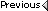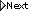» Online Classroom   » Tech Support   » Inland & Coastal Navigation Course   » Prob 6-1 answer

Author Topic: Prob 6-1 answer
 David Burch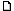posted January 27, 2005 06:44 PM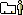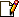The answer in the workbook is not right for this one. Here is correct answer.Race Rks light is 118 ft above MHW. Hence the total geo range is 1.15 x sqroot(118) + 1.15 x sqroot (9) = 12.49 + 3.45 = 15.94. Unfortunately, the factor of 1.15 will vary with the particular geographic range table. I believe the 1.15 is the latest best value. The nominal range is 15 on the chart and 18 on the light list edition we include. Visible range is always the smaller of the two, so if the chart is right the answer is 15 and if the Light List is right, the answer is 15.94.The Light List table we have appears to be using a factor of 1.17 (check out the value of H = 100 ft). And with those values, your solution is correct. Using 1.17 in the table we get (1.17/1.15)x15.94 = 16.22 which is roughly what you got interpolating the table.For the second, light ht is 67ft, so geo range (by table) = 1.17xroot(67) + 1.17xroot(9) = 9.58+3.45 = 13.03 From: Starpath, Seattle, WA

 All times are Pacific